## 大綱

• 安裝 Python 與 Jupyter
• Python 與資料科學
• Python 特色
• Python 環境架構與 Python Script
• Python 語言與資料科學
• Python 語法基礎
• 1.變數（variables）與資料型態、型別（types）
• 2.基本運算與是非邏輯（logic）
• 3.list、dict
• 4.if-else 陳述句
• 5.迴圈
• 6.函式（function）
• 7.檔案（File）
• 8.函式庫（libraries）
• 9.錯誤偵測（try catch）
• 10.物件導向（object）

## 安裝 Python 與 Jupyter

###### 什麼是 Jupyter Notebook

Jupyter 主要提供一個網頁介面，讓使用者可以透過瀏覽器撰寫程式，可以在上面進行 Python 、R 或 Scala 程式的開發！功能相當強大。其特色如下：

1. 適合開發資料科學相關的 Python 程式時使用，簡單的網頁編譯器，可以打完程式隨即執行
2. 可以用 Markdown 語法做豐富的筆記文件、寫數學式子、執行程式
3. 可以方便的看到用 Python 視覺化圖型的結果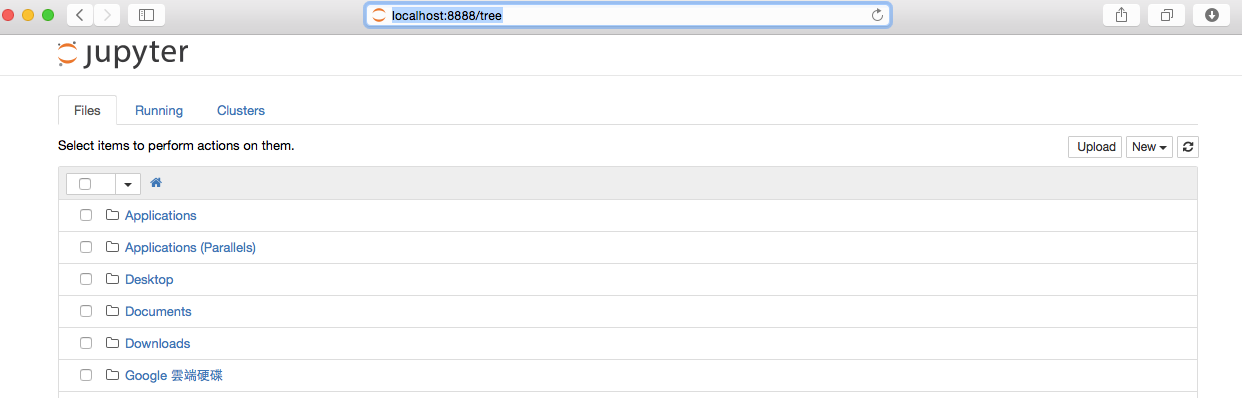## Python 與資料科學

###### Python 特色
1. 易寫、易讀、易學
2. 免費、開源，你可以自由的發布複製程式碼、閱讀它的原始碼、並把更動的部分用於新的開源軟件中
3. 跨平台，可以在這些平臺上執行，包括 Linux、Windows、MacOS 等
4. 擁有強大的社群以及生態系統
###### Python 環境架構與 Python Script
• Python 腳本以 .py 作檔案名稱結尾
• 內容包含的就是 Python 程式，也就是一連串的 Python 指令
• 通常會用自己偏好的文字編輯器來撰寫程式，例如：最新流行的 VSCode然後再執行它
• 可以在命令提示字元(cmd)輸入「python xxx.py」來執行 xxx.py 這個 python 腳本

``````python hello.py
``````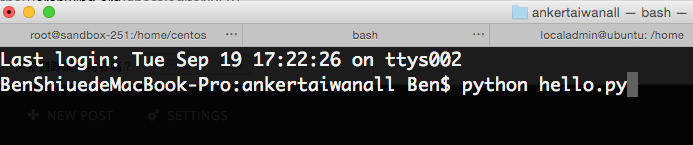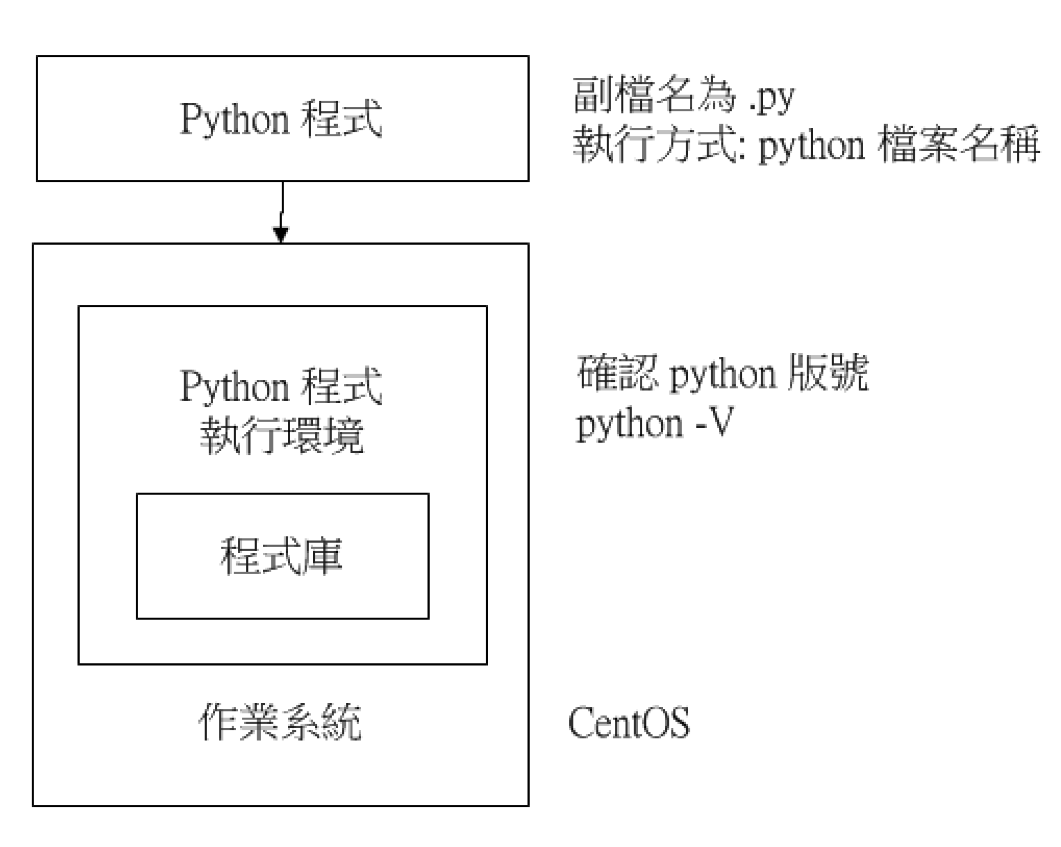###### Python 語言與資料科學

Python 本身就是拿來做做資料科學的應用？

• Python 本身並非被特地設計用來做資料科學應用
• Python 除了資料分析外，也常被拿來做網站後端，撰寫 API、IoT 開發板開發等應用
• Python 生態系統裡有很多第三方套件是專門為資料科學與科學計算而生

Python 常見做資料科學應用的套件：

• numpy：做向量矩陣轉換
• scipy：可以針對矩陣進行許多常見的統計計算
• pandas：做資料分析與資料處理
• matplotlib：做資料視覺化
• scikit-learn：做機器學習
• opencv：做影像辨識

## Python 語法基礎

###### 1.變數（variables）與資料型態、型別（types）
• 賦予名字給某個值
• 在程式裡重複使用
• 值可以不斷被改變
• 變數規則：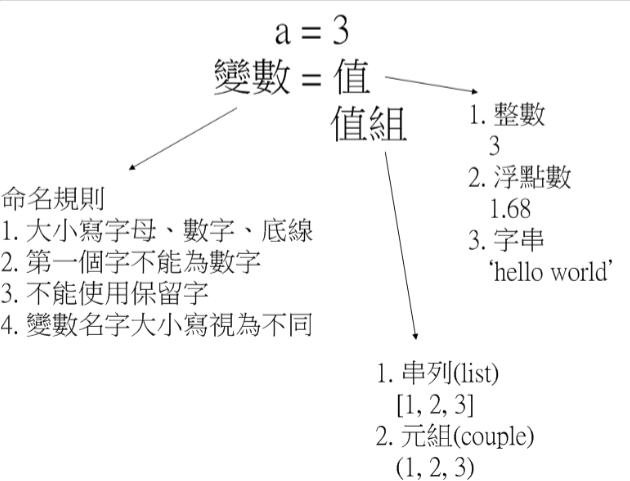❖ 變數名稱保留字：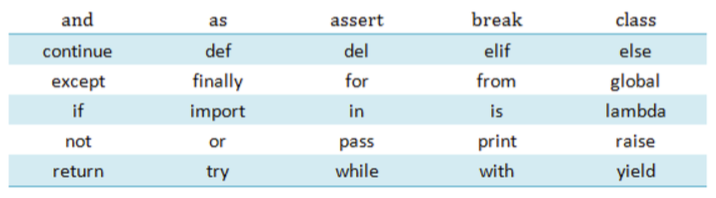❖ 指定變數的語法：

``````x = 20
``````

❖ 使用變數：

``````x * 40
``````

• 資料型態、型別（types）
• int：整數
• float：浮點數
• str：字串

❖ int：Integer，整數，沒有小數點

EX:

``````type(0)
type(-124819)
``````

Output 為：
int
int

❖ float：Float，浮點數，有小數點的數字

EX:

``````type(3.5)
``````

Output 為：
float

❖ str：String，字串，任何用單引號（'）或雙引號（"）括起來的文字都是字串

EX:

``````type('hello world')
type("你好")
type('3') #即使內容是數字，如果用引號括起來就是字串
``````

Output 為：
str
str
str

str 可以相加，會把兩個字串連在一起

``````'A' + 'Python'
``````

Output 為：
APython

###### 2.基本運算與是非邏輯（logic）
• 運算元、運算子
• 基本的加減乘除❖ 算數運算子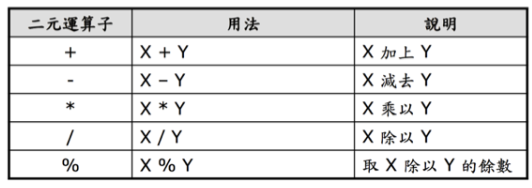EX:

``````print(5 + 3)
print(25 - 13)
print(5 * 3)
print(25 / 5)
``````

Output 為：
8
12
15
5

``````print(21 % 2) # 21 / 2 的餘數為 1，所以結果為 1
print(3 ** 5) # 3 的 5 次方
``````

Output 為：
1
243

• 比較運算子
• 如何判斷兩個數字是否相等？
• 兩串文字是否相等？
• 數字 A 是否大於數字 B？
• 我們可以用 == 來知道兩個值是否相等，用 != 來知道兩個值是否不相等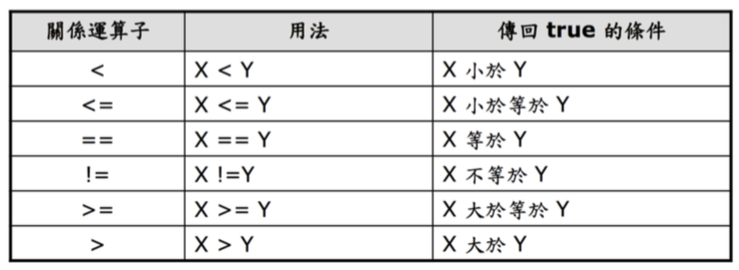EX：

``````print(2 == 2)
print(2 == 5)
print(3 != 3)
print(3 != 4)
``````

Output：
True
False
False
True

``````== 和 = 是完全不一樣的
= 用於指定，將左項指定為右項的值，左右不可交換
== 用於比較，比較兩者是否相等，左右可以交換
``````

``````print('word' == 'word')
print('word' != 'world')
``````

Output：
True
True

• 邏輯運算子
• 條件 X 成立「而且」條件 Y 成立
• 條件 X 成立「或者」條件 Y 成立
• 條件 X 「不成立」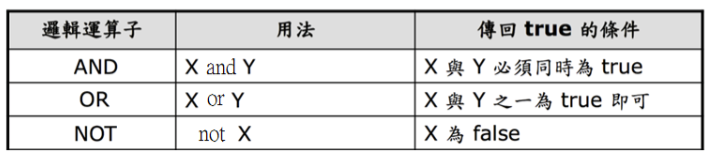EX：

``````print(True and True)
print(True and False)
print(True or False)
print(False or False)
print(not True)
print(not False)
``````

Output：
True
False
True
False
False
True

###### 3.list、dict

❖ List

EX：

``````a = ['Ben', 'Polo', 'Ken', 'Archer']
len(a)  #可以用 len 函式來檢視它的長度
a  #取得 list 中的元素
a[-1]  #從後面取得 list 中的元素
``````

Output：
4
'Ben'
'Archer'

1. a 是我們想檢視的 list
2. index 是從 0 開始而非 1
3. 因此 'Ben' 在這個 list 中的位置是 0 而非 1

EX：

``````a = ["1", "2", "3", "4", "5", "6", "7"]
print(a[3:6])
print(l[:5])
``````

Output：
['4', '5', '6', '7']
['1', '2', '3', '4']

1. 中括弧裡面的內容包含一個冒號：
2. 冒號前是開始位置
3. 冒號後是結束位置
4. 結果並不包含結束項

EX：

``````a = 'first'
print(a)
``````

Output：
['first', '2', '3', '4', '5', '6', '7']

EX：

``````a.append('eight')
print(a)
``````

Output：
['first', '2', '3', '4', '5', '6', '7', 'eight']

❖ Dictionary

1. 用一對大括弧 {} 來表示一個 dict 的開始與結束
2. 每一個元素都是一組 key-value
3. key 即是索引，每個索引對到一個值
4. key 與 value 中間用冒號隔開，每組之間用逗號隔開
5. 使用方法很類似 list，但 dict 是以 key 代表值的位置，因此我們可以用以下語法查看內容：

EX：

``````a_dict = {'name': 'Ben', 'height': 178}
print(a_dict['height'])

#增加資料到字典中
a_dict['age'] = 'xx'
print a_dict

#更新字典內的元素
a_dict.update({'height': 200})
print a_dict
``````

Output：
178
{'name': 'Ben', 'height': 178, 'age': 'xx'} {'name': 'Ben', 'height': 200, 'age': 'xx'}

``````# 遍歷整個字典資料
for rec in a_dict:
print rec, a_dict[rec]
``````

###### 4.if-else 陳述句

if 陳述句可以用來實現所謂的判斷流程，讓某些程式只有在某個條件成立時才會執行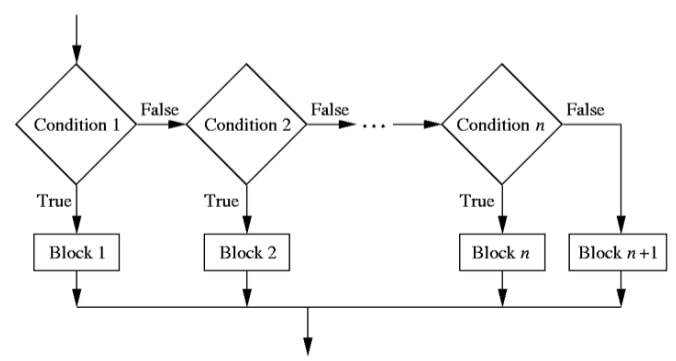if 語法需要注意的細節：

1. if 陳述句之後，必須加一個冒號
2. 只有在該判斷式成立時才會執行的程式碼，稱為 if 區塊（if block），if block 裡面的每一行程式碼，前面都必須縮排
3. else 後面也必須增加冒號，下面的內容叫 else block，else block 裡面的內容也必須縮排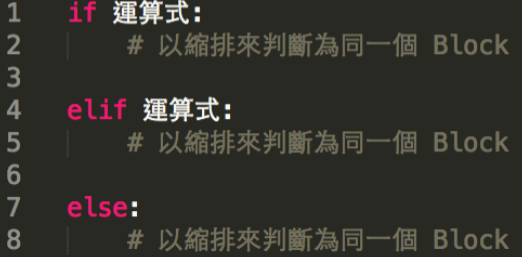EX：

``````num = input('please input a n')

if num%2 == 0:
print(num, 'even')
else:
print(num, 'odd')
``````
###### 5.迴圈

❖ for
for 迴圈擁有一個計數器，稱為迴圈變數。這使得 for 迴圈能夠知道過程中的執行順序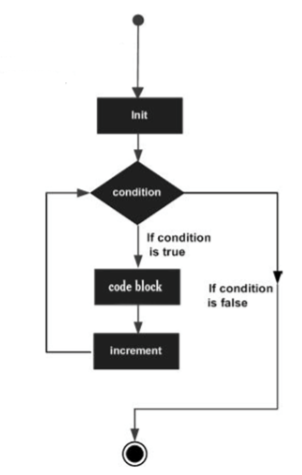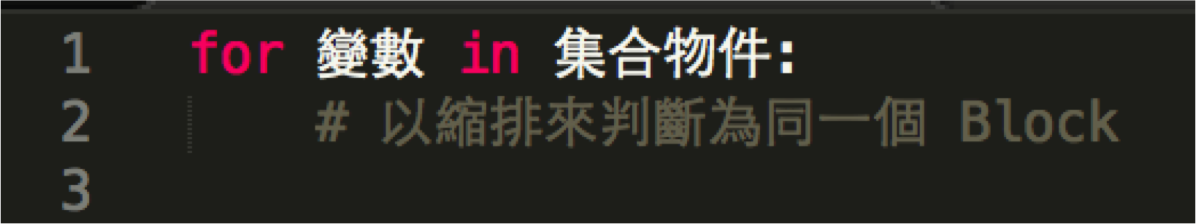EX：

``````no = [1,2,3,4,5,6,7,8,9]

for i in no:
print i
``````

Output：
1
2
3
4
5
6
7
8
9

❖ while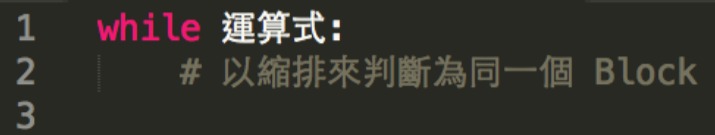EX：

``````# While 迴圈
x=0
while x < 10:
print 'x is currently: ',x
print 'x is still less than 10, adding 1 to x'
x+=1
else:
print 'All Done!'
``````

Output：
x is currently: 0
x is still less than 10, adding 1 to x
x is currently: 1
x is still less than 10, adding 1 to x
x is currently: 2
x is still less than 10, adding 1 to x
x is currently: 3
x is still less than 10, adding 1 to x
x is currently: 4
x is still less than 10, adding 1 to x
x is currently: 5
x is still less than 10, adding 1 to x
x is currently: 6
x is still less than 10, adding 1 to x
x is currently: 7
x is still less than 10, adding 1 to x
x is currently: 8
x is still less than 10, adding 1 to x
x is currently: 9
x is still less than 10, adding 1 to x
All Done!

###### 6.函式（function）

• print: 印出某個值
• len: 取得一個串列的長度
• type: 取得一個值的型別

EX：

``````len(list)
``````

5

1. len 是函式名稱
2. 函式名稱之後須加一對括弧，括弧間的是函式的輸入
3. 在這裡只有一個輸入，即 list
4. 若有多個輸入值，則用逗號分隔( , )
5. (非語法) 5 是這個函數呼叫的輸出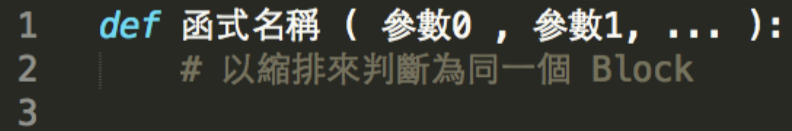EX：

``````# 函式
def addNum(a, b): # 函式命名標準會採取駝峰式的樣式
return a+b #Return
``````

Output：
5

``````# 接受多個 Key/Value 參數
def make_two_lists(**kwargs):
keys,values = [],[]
for k,v in kwargs.items():
keys.append(k)
values.append(v)
return [keys,values]

make_two_lists(david='M', Mary = 'F', John='M')
``````

Output：
[['John', 'Mary', 'david'], ['M', 'F', 'M']]

###### 7.檔案（file）

❖ 寫檔

EX：

``````#將Hello World 寫入檔案中
fid = open('test.txt', 'w')
fid.write('Hello\nWorld')
fid.close()
``````

❖ 讀檔

EX：

``````# 一行一行讀取檔案
with open('test.txt', 'r') as fid:
for line in fid:
print("Line: " + line.strip())

# 讀取檔案中所有內容
with open('test.txt', 'r') as fid:
print "讀取檔案中所有內容line =>",s
``````

Output：
Line: Hello
Line: World

###### 8.函式庫（libraries）

1. 前人或神人寫好的一堆函式
2. 使用者可以「引用」(import) 函式庫來使用這些函式
3. 讓程式碼更簡潔且開發更快速

EX：

``````# 引用函式庫，並顯示目前的時間
import time

# 我們可以使用這個函式庫裡面的函式strftime
proc_time = time.strftime("%Y-%m-%d %H:%M:%S")
print(proc_time)
``````

Output：
2017-09-21 06:51:11

EX：

``````# from 後面接的是函式庫名稱，import 後接的是函式名稱
from random import choice
choice([1, 5, 7, 3, 9, 0])
``````

Output：
5

###### 9.錯誤偵測（try catch）

EX：

``````# 處理 IO Exception
try:
f = open('testfile','w')
f.write('Test write this')
except IOError: #處理IOError
print("Error: Could not find file or read data")
else:
print("Content written succesfully")
f.close()
``````